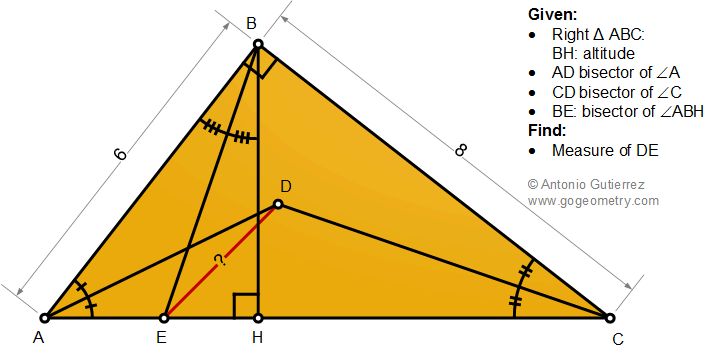# Geometry Problem 1487 with Solution: Right Triangle, Altitude, Angle Bisectors, Measurement

The figure shows a right triangle ABC with altitude BH. AD, CD, and BE are the bisectors of angles A, C, and ABH, respectively. If AB = 6, and BC = 8, find the measure of DE.